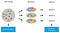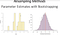# Bootstrapping

## Getting more out of what was given or currently have

By the end of this article, you will be able to:

• describe bootstrapping as a technique and explain how confidence intervals are computed using bootstrapping
• calculate bootstrapped correlations and confidence intervals using R
define a function in R
• calculate, interpret, and report partial correlation using R

Non-parametric tests

Last time, we saw another data set — a measure of how creative people are and their position (i.e., rank) in a “greatest liar” competition.

What data types are these?

Can we test the correlation between Creativity and Position? → not with Pearson!

Okay, so we have a model that requires assumptions and the data violate one or more assumptions …and the model is not robust to those violations. What can we do?

We can either 1) change the data, or 2) change the model

# Correlation measures

Pearson’s r

• Most common; requires interval data
• CIs or statistical tests require Normal sampling distribution (which you have if you have Normally distributed data, or a large sample size, e.g., >30)
• with one exception: one binary 0/1 variable is okay)

Spearman’s rho

• Non-parametric
• Conducts rank transform (ranks all data), then calculates Pearson’s

Kendall’s tau

• non-parametric
• preferred when there are a lot of tied ranks

But, there’s one more way of dealing with assumptions more generally.

# Bootstrapping

Bootstrapping is a statistical procedure that resamples a single dataset to create many simulated samples. This process allows you to calculate standard errors, construct confidence intervals, and perform hypothesis testing for numerous types of sample statistics.Kaggle

The assumption is that the sample population is similar to the general population

The procedure is randomly resampled from the sample with replacement to produce estimates of your test statistic. You take the mean as your estimate and 95% quantiles for a 95% confidence interval. This method is good when there’s no closed-form solution for confidence intervals, etc.

Let’s watch a video: https://www.youtube.com/watch?v=gcPIyeqymOU

The boot library can be used to do bootstrapping.

If you want to measure the height of a population then you take a sample (since you cannot measure everyone in the population. You can calculate the mean of the population and continue sampling and sampling until you get a normal distribution. Usually, the sampling distribution follows a normal distribution and can calculate SD and CI from it. Instead of grabbing a bunch of different samples (because it is expensive and time-consuming), you can get a sample and resample that sample. Imagine if you have a bag of marbles, grab a marble, put it back in, and repeat to create a sample and do it multiple times. This will give you a sampling distribution.

Every time you sample (the same size, resample 20 heights with replacement to get the variance. If not, you will get the same person every time), you treat it as a sampling distribution. This helps estimate the entire population from a single sample to estimate parameters.Eart125: Statistics and Data Analysis in Geosciences — UC Santa Cruz

You can calculate confidence intervals using `boot.ci()`

This takes the quantiles (e.g., of the middle 95\%) to calculate the confidence intervals.

Bootstrapping is a very general technique — you can use it for pretty much anything.

Because of this, you need to write a function to define what you’d like the bootstrap function to calculate at each sample.

Let’s try this on a function:

Use bootstrapping to calculate an estimate of Spearman’s correlation coefficient of Creativity and Position, and report the 95\% confidence intervals.

What does bootRho do?

Every time you use bootRho, you get to 2 arguments: you have a dataset (which can be any dataset and is liarData in this case) and i (which is the resampled indices, in this case, you have 1 1 3 10 8 22, whatever up to 68 and it is the resampling to help you get the values).

So now we can take a look at the usage called bootSpearmanResult, the boot would take in data (liarData, the function bootRho which will calculate based on the resample, and the number of replicates R which is 2000). We get this function passed through the dataset 2000 times and resample the i based on the data. It essentially calls bootRho 2000 times, which why we had to define it.

As a result, we have lots of things going. The t-value is what we are calculating. There will be 2000 values and they are correlation coefficients. Each value produced is the correlation coefficient from the resampling. Data will converge into a single stationary value then we won’t need any more resampling. The resampling size doesn’t affect much.

→ 2000 times, it will grab 68 samples randomly then calculate the correlation.

# Key Takeaways

Assumptions can be circumvented by resampling

• Bootstrapping is a general technique to produce estimates and confidence intervals of various statistics
• This makes a single assumption: that your sampled population is a good representation of the general population

Data science and Blockchain Enthusiast | Chess Player

Data science and Blockchain Enthusiast | Chess Player